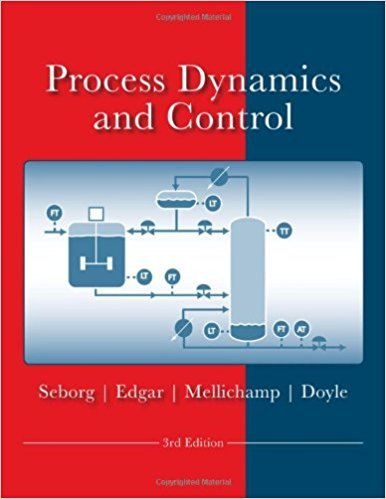×
Get Full Access to Process Dynamics And Control - 3 Edition - Chapter 3 - Problem 3.1
Get Full Access to Process Dynamics And Control - 3 Edition - Chapter 3 - Problem 3.1

×

# The differential equation (dynamic) model for a chemicalISBN: 9780470128671 148

## Solution for problem 3.1 Chapter 3

Process Dynamics and Control | 3rd Edition

• Textbook Solutions
• 2901 Step-by-step solutions solved by professors and subject experts
• Get 24/7 help from StudySoup virtual teaching assistantsProcess Dynamics and Control | 3rd Edition

4 5 1 314 Reviews
30
3
Problem 3.1

The differential equation (dynamic) model for a chemical process is as follows: d2y dy - + 5- + 3y = 2u(t) dil dt where u(t) is the single input function of time. y(O) and dyldt (0) are both zero. What are the functions of the time (e.g., e-tiT) in the solution to the ODE for output y(t) for each of the following cases? (a) u(t) = be - 21 (b) u(t) = ct b and c are constants. Note: You do not have to find y(t) in these cases. Just determine the functions of time that will appear in y(t).

Step-by-Step Solution:
Step 1 of 3

Predicting bond polarity Chemical bonds are usually polar whenever the two atoms involved have different electronegativities, because the more electronegative atom will tend to pull the shared valence electrons in the bond toward it. Since electrons have a negative charge, that will give the more electronegative atom a slight negative charge. The other atom will get a slight positive charge, because it will no longer have enough electrons to fully cancel the positive charge of its nucleus. • The higher the electronegativity, the more negative the element (when comparing bond polarity) Note: The polarity is only noticeable in practice when the difference in electronegativities between atoms is greater than about 0.50. Your instructor may want you to label bonds between ato

Step 2 of 3

Step 3 of 3

##### ISBN: 9780470128671

This textbook survival guide was created for the textbook: Process Dynamics and Control, edition: 3. Since the solution to 3.1 from 3 chapter was answered, more than 1002 students have viewed the full step-by-step answer. The full step-by-step solution to problem: 3.1 from chapter: 3 was answered by , our top Engineering and Tech solution expert on 11/15/17, 04:03PM. Process Dynamics and Control was written by and is associated to the ISBN: 9780470128671. The answer to “The differential equation (dynamic) model for a chemical process is as follows: d2y dy - + 5- + 3y = 2u(t) dil dt where u(t) is the single input function of time. y(O) and dyldt (0) are both zero. What are the functions of the time (e.g., e-tiT) in the solution to the ODE for output y(t) for each of the following cases? (a) u(t) = be - 21 (b) u(t) = ct b and c are constants. Note: You do not have to find y(t) in these cases. Just determine the functions of time that will appear in y(t).” is broken down into a number of easy to follow steps, and 100 words. This full solution covers the following key subjects: cases, functions, function, chemical, constants. This expansive textbook survival guide covers 24 chapters, and 420 solutions.

Unlock Textbook Solution